With the stock up 18.8% over the prior month, investors may be tempted to sell their shares of TreeHouse Foods Inc (NYSE: THS). In this article, I am going to calculate the fair value of TreeHouse by forecasting its future cash flows and discounting them back to today’s value. Value investors may find the results from my analysis surprising.

DCF Methodology

The basic philosophy behind a DCF analysis is that the intrinsic value of a company is equal to the future cash flows of that company, discounted back to present value. The general formula is provided below. The intrinsic value is considered the actual value or “true value” of an asset based on an individual’s underlying expectations and assumptions.Cash flows into the firm in the form of revenue as the company sells its products and services, and cash flows out as it pays its cash operating expenses such as salaries or taxes (taxes are part of the definition for cash operating expenses for purposes of defining free cash flow, even though taxes aren’t generally considered a part of operating income). With the leftover cash, the firm will make short-term net investments in working capital (an example would be inventory and receivables) and longer-term investments in property, plant and equipment. The cash that remains is available to pay out to the firm’s investors: bondholders and common shareholders.

I will take you through my own expectations for TreeHouse as well as explain how I arrived at certain assumptions. The full analysis was completed on Thursday, May 10. An updated analysis using real-time data can be viewed in your web browser at finbox.io’s TreeHouse DCF analysis page. The steps involved in the valuation are:

1. Forecast Free Cash Flows
• Create a revenue forecast
• Forecast EBITDA profit margin
• Calculate free cash flow

Step 1: Forecast Free Cash Flows

The key assumptions that have the greatest impact on cash flow projections are typically related to growth, profit margin and investments in the business. The analysis starts at the top of the income statement by creating a forecast for revenue and then works its way down to net operating profit after tax (NOPAT), as shown below.

Revenue - Operating Expenses = EBIT - Taxes = NOPAT

NOPAT - Capital Expenditures - NWC Investment + D&A = Unlevered FCF

From NOPAT, deduct cash outflows like capital expenditures and investments in net working capital and add back non-cash expenses from the income statement such as depreciation and amortization to calculate the unlevered free cash flow forecast (shown above).

Create A Revenue Forecast

When available, the finbox.io’s pre-built models use analyst forecasts as the starting assumptions. To forecast revenue, analysts gather data about the company, its customers and the state of the industry. I typically review the analysts’ forecast and modify the growth rates based on historical performance, news and other insights gathered from competitors. Note that if a company only has a small number of analysts giving projections, the consensus forecast tends to not be as reliable as companies that have several analysts’ estimates. Another check for reliability is to analyze the range of estimates. If the range is really wide, it may be less accurate.

TreeHouse Foods operates as a food and beverage manufacturer in the United States, Canada, and Italy. Analysts covering the stock often compare the company to a peer group that includes Campbell Soup Company (NYSE: CPB), ConAgra Brands, Inc. (NYSE: CAG), Hershey Company (The) (NYSE: HSY), and J.M. Smucker Company (The) (NYSE: SJM).source: Benchmarks: Forecasted 5yr Revenue CAGRs

The company’s projected 5-year revenue CAGR of -0.7% is below all of its selected comparable public companies: CPB (0.3%), CAG (1.0%), HSY (2.9%) and SJM (0.7%).

As highlighted below, TreeHouse’s revenue growth has ranged from 2.1% to 92.6% over the last five fiscal years.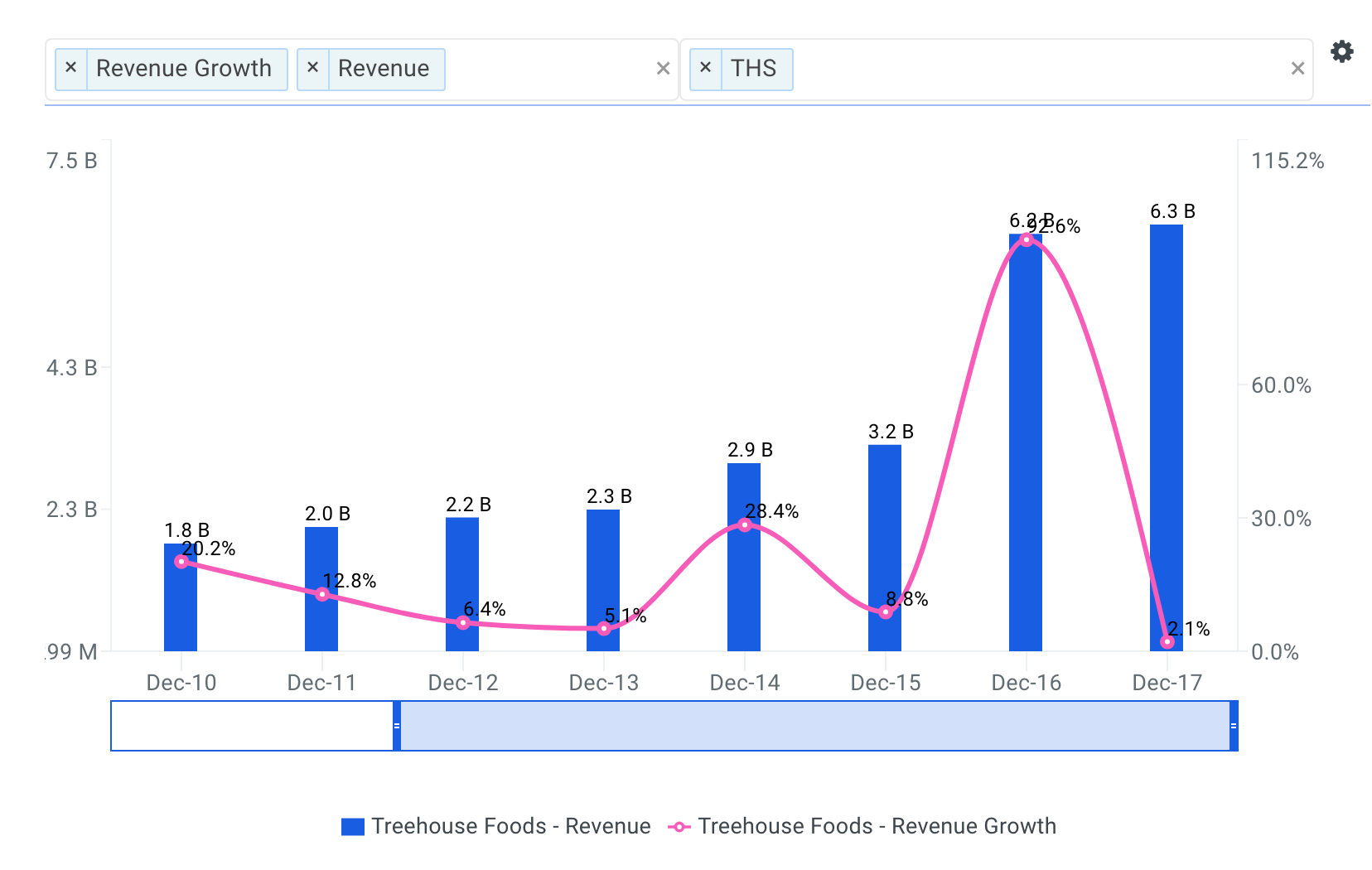Going forward, analysts forecast that TreeHouse’s total revenue will reach \$6,084 million by fiscal year 2022 representing a five-year CAGR of -0.7%.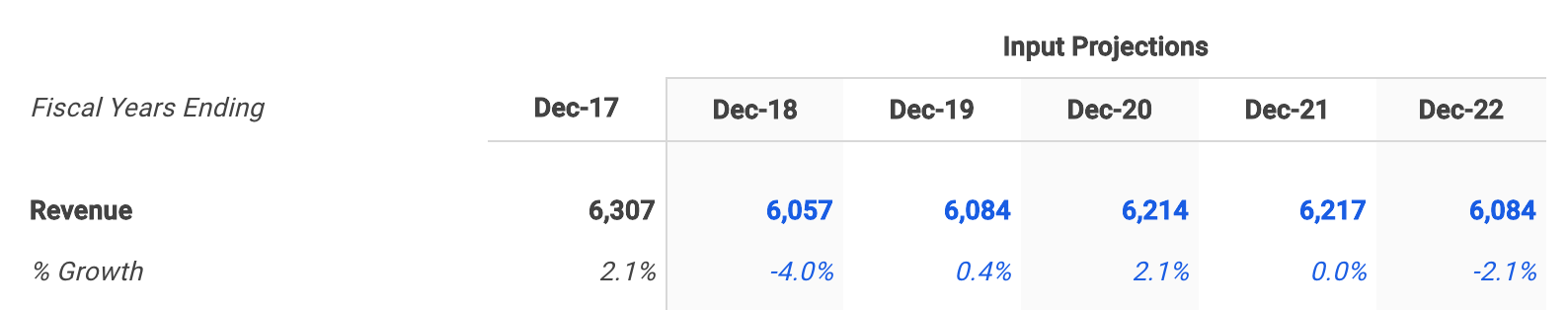Forecast TreeHouse’s EBITDA Profit Margin

The next step is to forecast the company’s earnings before interest, taxes, depreciation and amortization (EBITDA). Note that EBITDA is a commonly used metric in valuation models because it provides a cleaner picture of overall profitability, especially when benchmarking against comparable companies. This is because it ignores non-operating costs that can be affected by certain items such as a company’s financing decisions or political jurisdictions. For more detail, see TreeHouse’s EBITDA definition.

EBITDA margin is calculated by dividing EBITDA by revenue. The higher the EBITDA margin, the smaller the firm’s operating expenses are in relation to its revenue, which may ultimately lead to higher profit. Lower operating expenses for a given level of revenue can be a sign of internal economies of scale.

The charts below compare TreeHouse’s LTM EBITDA margin to the same peer group. The company’s EBITDA margin of 6.8% is below all of its selected comparable public companies: CPB (21.9%), CAG (16.3%), HSY (23.2%) and SJM (18.2%).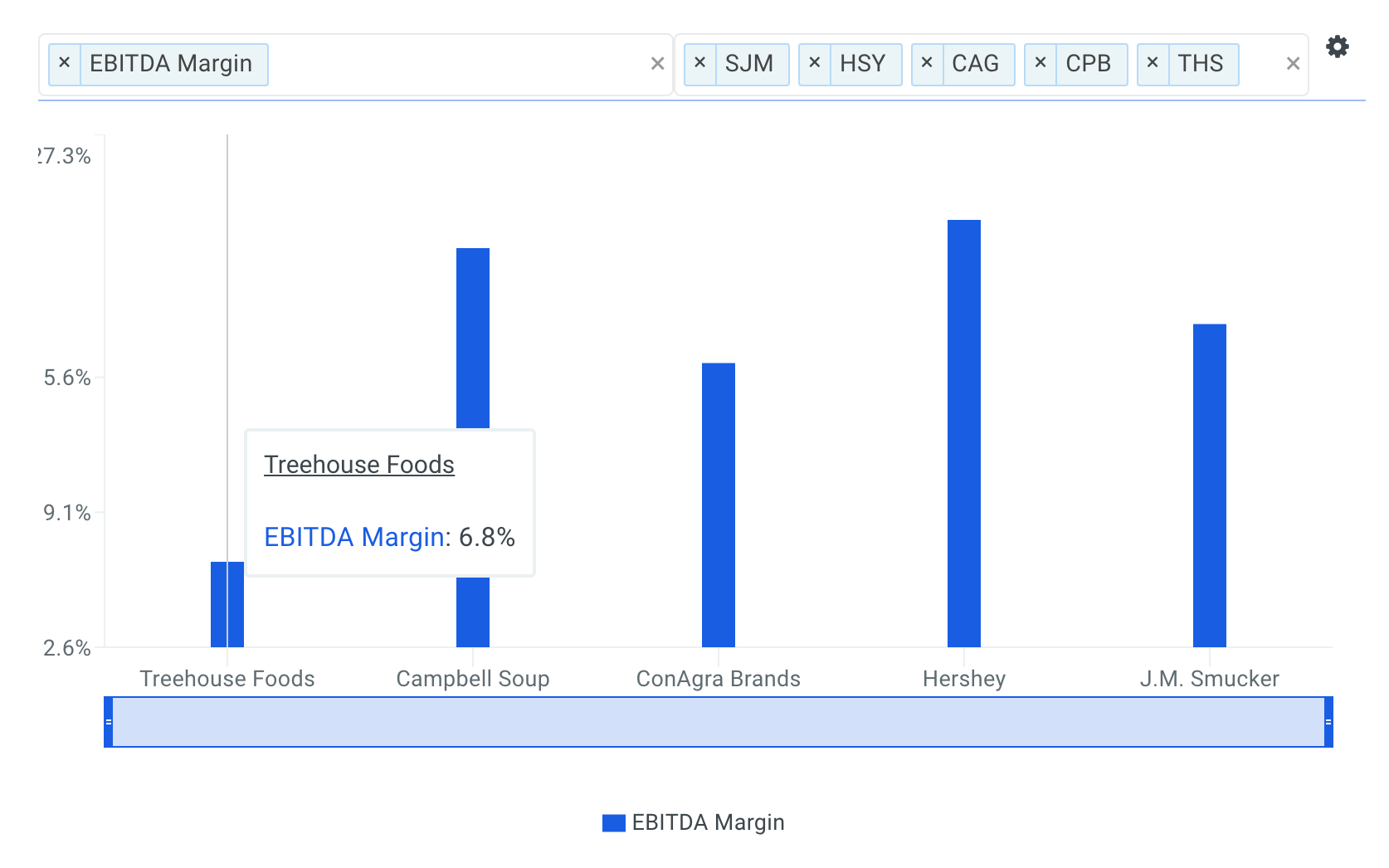TreeHouse’s EBITDA margin has ranged from 6.8% to 12.5% over the last five fiscal years.Wall Street analysts are forecasting that TreeHouse’s EBITDA margin will reach 10.2% by fiscal year 2022, representing an increase of 3.4% from its LTM EBITDA margin of 6.8%.Although I don’t specifically walk through my assumptions here, I then forecasted depreciation & amortization, capital expenditures and net working capital based on historical levels.

Calculate Free Cash Flow

With all required forecasts in place, the next step is to calculate projected free cash flow as shown below.Step 2: Select TreeHouse’s Discount Rate

The next step is to select a discount rate to calculate the present value of the forecasted free cash flows. I used finbox.io’s Weighted Average Cost of Capital (WACC) model to help arrive at an estimate. Generally, a company’s assets are financed by either debt (debt is after tax in the formula) or equity. WACC is the average return expected by these capital providers, each weighted by respective usage. The WACC is the required return on the firm’s assets.

It’s important to note that the WACC is the appropriate discount rate to use because this analysis calculates the free cash flow available to TreeHouse’s bondholders and common shareholders. On the other hand, the cost of equity would be the appropriate discount rate if we were calculating cash flows available only to TreeHouse’s common shareholders (i.e., dividend discount model, equity DCF). This is commonly referred to as the difference between free cash flow to equity (FCFE) and free cash flow to the firm (FCFF). By using the WACC to discount FCFF, we are calculating total firm value. If we discounted FCFE at the required return on equity, we would end up with equity value of the firm. Equity value of the firm is simply total firm value minus the market value of debt.

I determined a reasonable WACC estimate for TreeHouse to be 7.0% at the midpoint. An updated cost of capital analysis using real-time data can be found at finbox.io’s TreeHouse WACC Model Page. The DCF model then does the heavy lifting of calculating the discount factors by applying the mid-year convention technique.

Step 3: Estimate TreeHouse’s Terminal Value

Since it is not reasonable to expect that TreeHouse will cease its operations at the end of the five-year forecast period, we must estimate the company’s continuing value, or terminal value. Terminal value is an important part of the DCF model because it accounts for the largest percentage of the calculated present value of the firm. If you were to exclude the terminal value, you would be excluding all the future cash flow past the horizon period. Using finbox.io, users can choose a five-year or 10-year horizon period to forecast future free cash flow.

The most generally accepted techniques to calculate a terminal value are by applying the Gordon growth approach, using an EBITDA exit multiple and using a revenue exit multiple. This analysis applies the Gordon growth formula: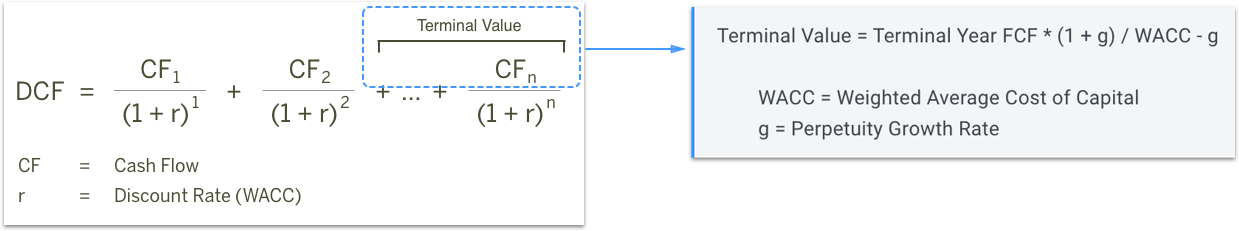As the formula suggests, we need to estimate a “perpetuity” growth rate at which we expect TreeHouse’s free cash flows to grow forever. Most analysts suggest that a reasonable rate is typically between the historical inflation rate of 2% to 3% and the historical GDP growth rate of 4% to 5%.

TreeHouse’s free cash flows are still growing at the end of the projection period, so I’ve selected a perpetuity growth rate of 1.0% (at the midpoint).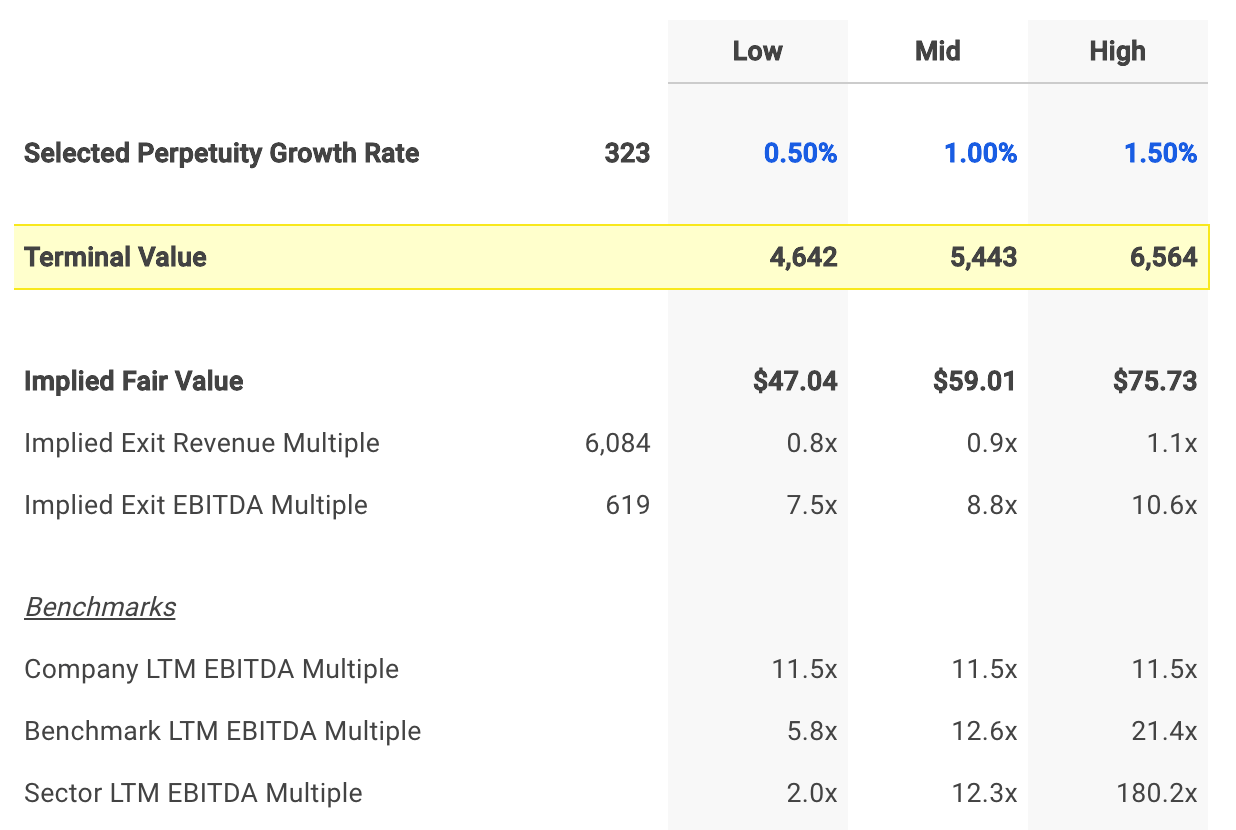Step 4: Calculate TreeHouse’s Equity Value

The enterprise value previously calculated is a measure of the company’s total value. An equity waterfall is a term often used by valuation firms, referring to the trickle-down process of computing a company’s equity value from its enterprise value. Note that in the event of a bankruptcy, debt holders will be paid in full before anything is distributed to common shareholders. Therefore, we must subtract debt and other financial obligations to determine a firm’s equity value. The general formula for calculating equity value is illustrated in the figure below.

Enterprise Value - Debt - Minority Interest & Other Liabilities - Preferred Equity - Pension + Cash & Equivalents = Total Equity Value

The model uses the formula shown above to calculate equity value and divides the result by the shares outstanding to compute intrinsic value per share as shown at the bottom of the figure below.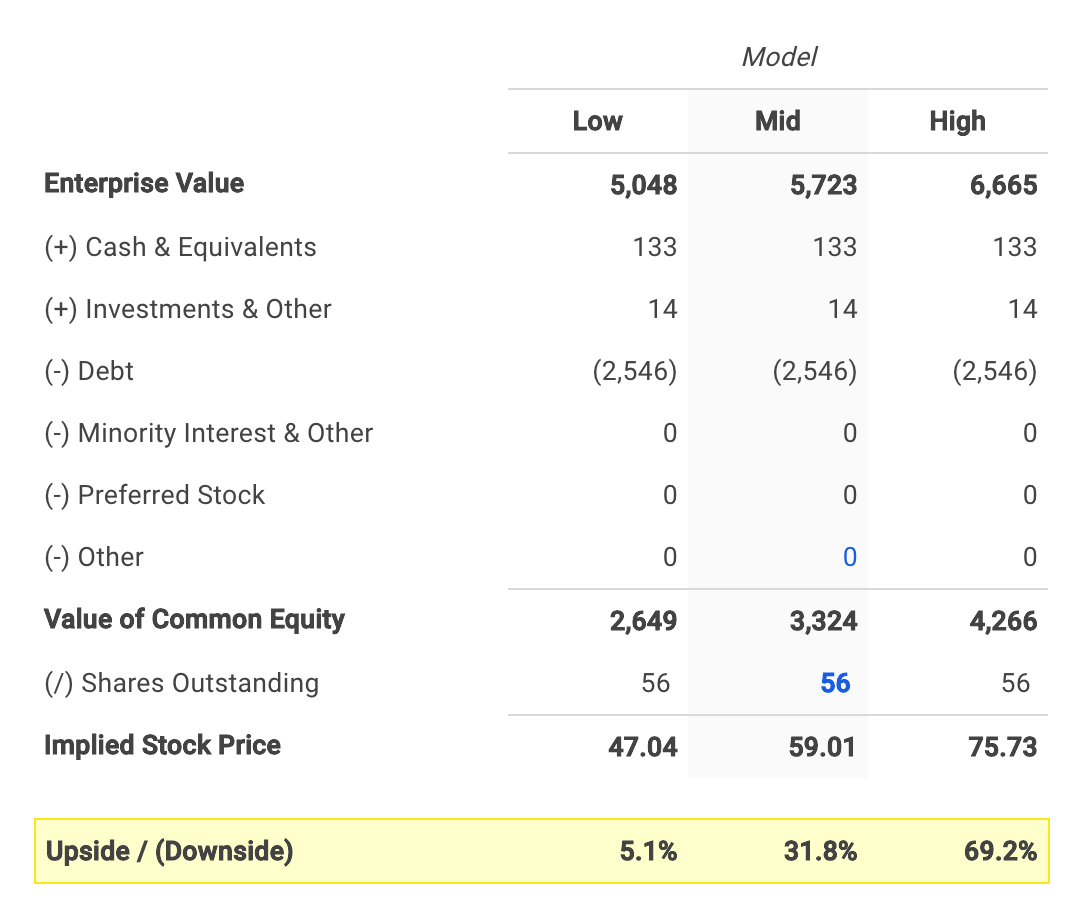The assumptions I used in the model imply an intrinsic value per share range of \$47.04 to \$75.73 for TreeHouse.

TreeHouse’s stock price currently trades at \$46.69 as of Thursday, May 10, 31.8% below the midpoint value of \$59.01.

Conclusion: TreeHouse Has Upside Potential

A DCF analysis can seem complex at first, but it’s worth adding to your investment analysis toolbox since it provides the clearest view of company value.

TreeHouse’s stock has made impressive gains over the prior month and investors may very well decide to sell their shares. However, the stock still appears to have further upside potential based on its future cash flow projections.

A DCF analysis is only one side of the coin in terms of building your investment thesis, and it shouldn’t be the only tool you use when researching a company. I recommend you continue to research TreeHouse to get a more comprehensive view of the company by looking at:

Valuation Metrics: how much upside do shares of Treehouse have based on the Ben Graham Formula? Take a look at our Ben Graham Formula data explorer which also compares the company’s upside to its peers.

Risk Metrics: what is Treehouse’s Altman Z score? It’s a famous formula used to predict the probability that a firm will go into bankruptcy within two years. View the company’s Altman Z score here.

Efficiency Metrics: how much free cash flow does Treehouse generate as a percentage of total sales? Has it been increasing or decreasing over time? Review the firm’s free cash flow margin here.

This is not a buy or sell recommendation on any security mentioned.

Expertise: financial modeling, mergers & acquisitions. Andy is also a founder at finbox.io, where he’s focused on building tools that make it faster and easier for investors to do investment research. Andy’s background is in investment banking where he led the analysis on over 50 board advisory engagements involving mergers and acquisitions, fairness opinions and solvency opinions. Some of his board advisory highlights: - Sears Holdings Corp.’s \$620 mm spin-off via rights offering of Sears Outlet, Hometown Stores and Sears Hardware Stores. - Cerberus Capital Management’s \$3.3 bn acquisition of SUPERVALU Inc.’s New Albertsons, Inc. assets. Andy can be reached at andy@finbox.io or at +1 (516) 778-6257.

This site uses Akismet to reduce spam. Learn how your comment data is processed.